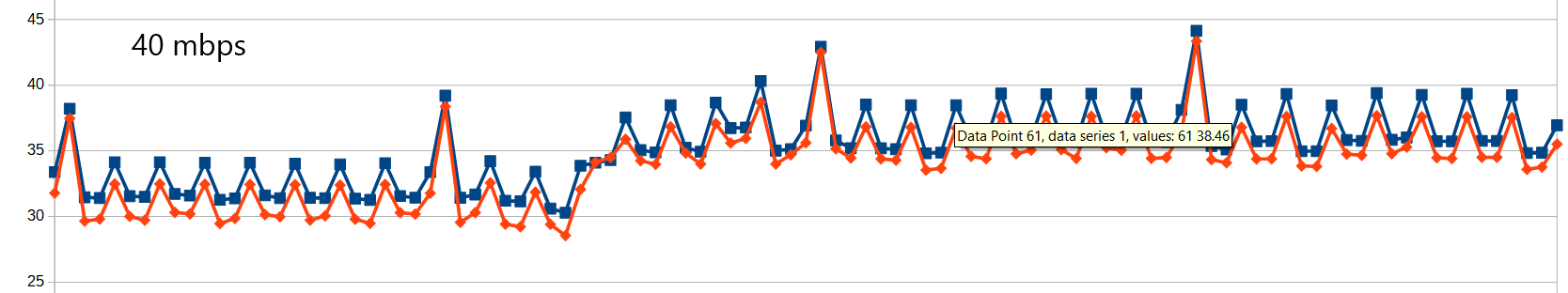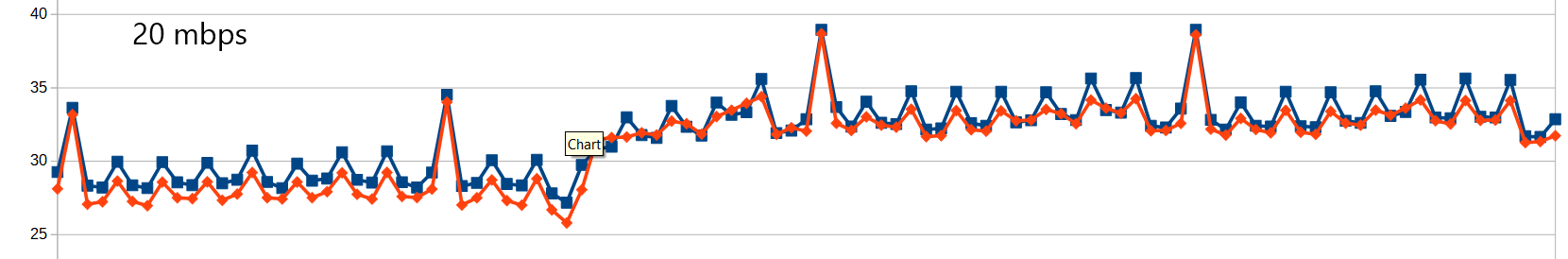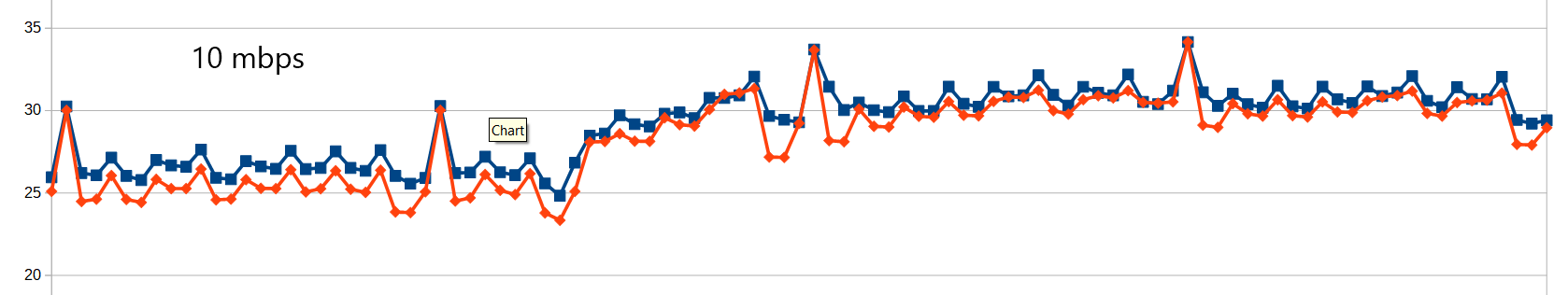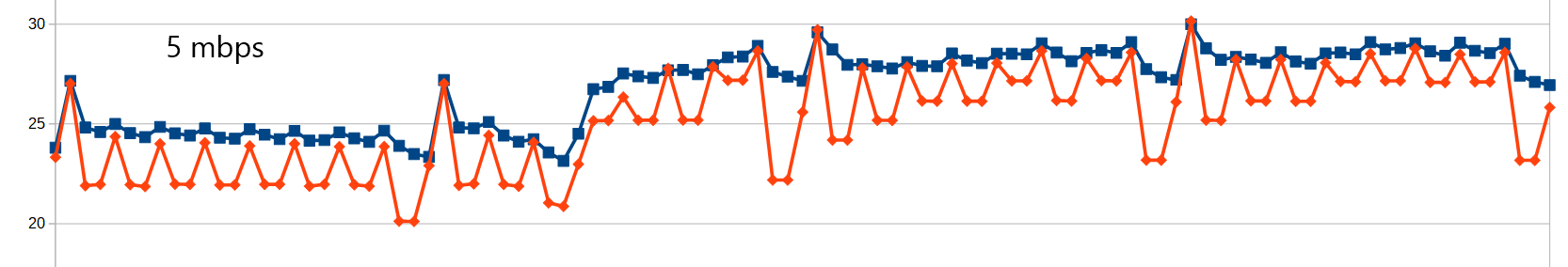# EPSNR metric

EPSNR metric

## Elecard EPSNR metric

EPSNR – Estimated Peak to Signal Ratio is a metric, developed in Elecard in order to estimate how much video was affected during the last compression in case of absence of source video.

EPSNR measures a relative size of quantizer to quantized DCT coefficient expressed in db, so could be compared with PSNR. Say, coefficient in the encoded stream is equal to 5 and quantizer is 20. Thus, de-quantized coefficient will be equal to 100, and source coefficient, before quantization, was somewhere in the range from 90 to 110. If a coefficient is equal to zero after quantization, the original value lays somewhere in the range -10 – +10 (for Q=20).

So, if we modify all de-quantized coefficients adding to them a random number from the range -Q/2 – +Q/2 it will correspond with the real quantization error. Of course, it’s not true for small coefficients from the lower right side of the transformed block, since its distribution is not even, but closer to Gaussian distribution around a zero. So, special modifications are applied for smaller coefficients.

Coefficients, modified with above approach are used to calculate pixels, then the standard PSNR is calculated between the decoded image and modified one. Resulting EPSNR curve behave similar to real PSNR.

It is evident that this method works better with higher coefficients and lower quantizers, i.e. for high motion, detailed video encoded at high bitrates.

Below, you may see a few samples of actual PSNR and EPSNR metrics calculated for the same video with different compression ratio.Blue – PSNR, red – EPSNR. Scale in db. Video – 1080p, has a lot of small details, extremely hard to encode.
You may see, that model works fine for 10-40 mbps, while at 5 mbps has significant problems with B-frames. I- and P-frames are more or less good, even at 5 mbps at PSNR’s of 25-27 db. This is due to mentioned problem of small coefficients. For the test video at 5 mbps almost all coefficients in B-frames are equal to zero, and it’s hard to estimate its real values distribution. Average size of compressed B-frames at 5 mbps is 4,170 bytes, while P-frames – 45,729 – this difference gives such a big error in EPSNR calculation for B-frame in comparison to P-frame.

October 13, 2017